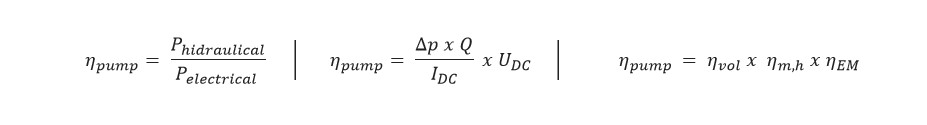# Performance parameters

## Performance Parameters of COR Pump

The sources of performance loss in a COR pump are divide into two sections: mechanical-hydraulic loses and volumetric loses. Mechanically hydraulic losses arise due to friction in the gaps, friction caused by turbulent liquid flows and friction from the difference in pressure in the system. Volumetric losses include losses due to internal leakage, and loss due to the compressibility of the pumping fluid.

The purpose of hydraulic pumps is to convert mechanical energy into hydraulic energy. Efficiency characteristics are the parameter-defining ratio between input energy and useful energy on the exiting side of the pump system. The hydraulic power is defined as the product of torque value and angular velocity on the shaft, which drives the pump rotor: the product of pressure difference (output-input) and the flow of liquid. The overall efficiency is defined based on the conversion of mechanical work into hydraulic power.In the assembly, when the electric motor is part of the pump, the total efficiency of the system is defined as the ratio between hydraulic power on input and electrical power given from e-motor. Electric power is equal to the product of the DC current and the electrical voltage UDC. Total efficiency of pump is given as a combination of electrical current and voltage, pressure difference and liquid flow. The total efficiency of the pump can also be defined as product of volumetric efficiency, mechanical-hydraulic efficiency and efficiency of e-motor.Leakages within pump unit relate to the size of gaps between pump elements, pressure difference (input vs. Output), rotation speed, volumetric displacement and viscosity of the fluid. Volumetric efficiency is defined as the ratio between real output flow and theoretical volumetric flow defined by pump ideal geometry. In positive displacement pumps, flow of liquid is linearly correlated to rotation speed.

The internal leakage is one of the most significaant sources of pump efficiency losses, through which the geometry is strongly influenced by the manufacturing process. It appears between the 3D shapes of pump parts and in the air gap in journal bearing of e-motor.